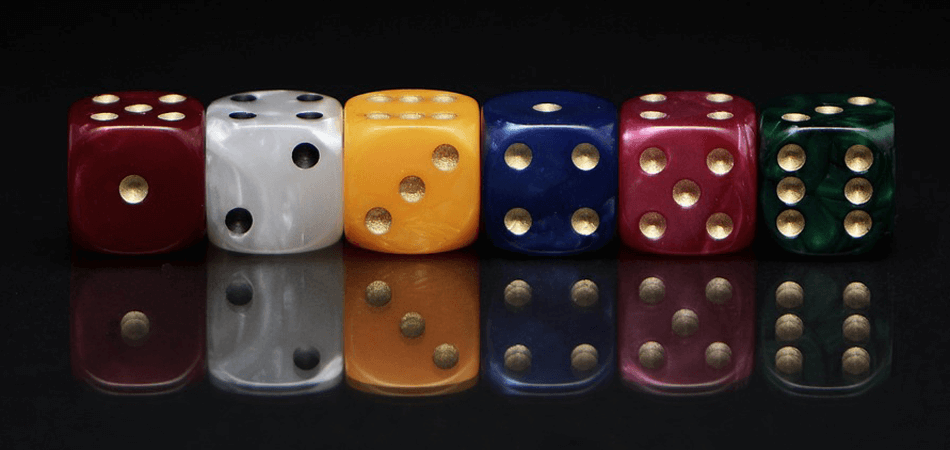# Lottery Mathematics – Probability, Permutations and CombinatoricsThere are countless strategies for picking lottery numbers of which most involve spurious methodology. While some claim to have found a magic formula that guarantees results, the fact is that lotteries are all about pure chance. Apart from actually buying a ticket or purchasing every single number combination, it’s just not possible to significantly improve one’s odds.

This hard truth is supported by the theories of probability, combinations and permutations. These established branches of mathematics allow us to accurately calculate the ludicrous odds inherent in lottery play.

### Probability

In brief, probability tells us how likely an event is to occur. It is always a value of between 0 (0%) and 1 (100%) with 0 representing impossibility and 1 indicating certainty. A random event is therefore more likely to happen if its probability is closer to 1 and less likely if it’s closer to 0. It is calculated using the following formula.

Probability =

number of ways an event can occur
total number of possible outcomes

So in applying this equation to calculate the probability of throwing a 7 with a die, it’s clear that the event can only occur once because there’s just a single instance of 7 on the die. The six sides of the die represent the total number of possible outcomes. Therefore:

P (7) = 1/6 = 0.167 (16.7%)

If there are two dice, the probability of throwing a 7 with the first die and then an 8 with the other is calculated like so:

P (7) = 1/6
P (8) = 1/6
P (7) x P (8) = 1/6 x 1/6 = 1/36 = 0.028 (2.8%)

In relation to the lottery then, the probability of winning is denoted as follows:

Probability of winning the lottery =

Amount of winning lottery numbers
Total number of possible lottery numbers

To work out the amount of winning lottery numbers and the total number of possible lottery numbers we need to consider combinatorics.

### Combinatorics

Combinatorics is a branch of mathematics concerned with sets of objects and the various ways in which their subsets and elements can be counted. As such, it is well-suited to calculating lottery odds. In order to apply combinatorics, we’ll take as an example the UK lotto. This type of lottery game follows a 6/59 format; that is, six numbers are drawn from a pool of 59 balls.

If the six numbers on a ticket match the six drawn numbers, the ticket holder wins a jackpot. To work out the odds of winning, we need to apply combinatorics because there is no particular order in which the winning numbers need to appear. If there was a set order, then the theory of permutations would come into play and the following combinatorial formula used:

n!/r!(n-r)!

This is the variant of the permutations and combinations formula and relates to unordered events. The letter n represents the total amount of possible numbers while represents the total amount of numbers chosen. The symbol ! is a factorial which means that it’s the product of an integer.

e.g. 6! = 6 x 5 x 4 x 3 x 2 x 1.

So for the UK Lotto, there are 59 lottery balls, all numbered individually. And there are 59 different, but equally likely ways of choosing the number of the first ball drawn from the pool of 59. Therefore, there is a 1 in 59 chance of predicting a single number correctly.

For the second number, there now remain 58 balls because the previous ball is not returned, which leaves a 1 in 58 chance of correctly choosing this number. The chances of matching a third drawn number are 1 in 57 and so on. This continues until finally, the sixth number is drawn. Consequently the final calculation is 49 × 48 × 47 × 46 × 45 × 44 = 6!, which can also be expressed thus:

59!/(6!(59-6)!) or 59!/((59-6)!)= 32,441,381,280

The number of times a set of six numbers can be arranged is then calculated. As we have already seen, this is worked out the following way:

6! = 6 x 5 x 4 x 3 x 2 x 1 = 720

Finally, by dividing 32,441,381,280 by the 720 orders we arrive at the number 45,057,474 which is also written as:

59!/((6!x(59-6)!) )

Therefore the odds of picking 6 correct numbers in the UK Lotto are 1 in 45,057,474

### COMBIN Formula

Working out factorials on a calculator can become rather complicated given the size of numbers often involved. To get around this, Excel has a built-in function known as the COMBIN Formula. Categorised under the Math/Trig drop-down menu, it returns the number of combinations for a specified number of items which is very useful for calculating lottery odds. It can be easily entered as part of a formula or cell in a worksheet. The syntax is:

COMBIN (number,chosen)

Although the chances of winning the UK lottery jackpot are miniscule, it’s worth pointing out that under new rules, should the jackpot exceed £50 million, the money has to be paid out in the next draw, regardless of whether a ticket matches all six balls. And if nobody picks the right numbers, the next best ticket wins. So there’s no harm in buying a ticket in scenarios like this – in fact your odds will improve slightly.

But playing the lottery on a regular basis as some kind of investment strategy is pure folly – the odds are simply too great. The only way to have any chance of making a meaningful investment is to spend millions on the bulk-buying of tickets. Even this is a risky and unpredictable tactic. A better approach would be to see the lottery for what it is: a form of entertainment to be enjoyed occasionally.